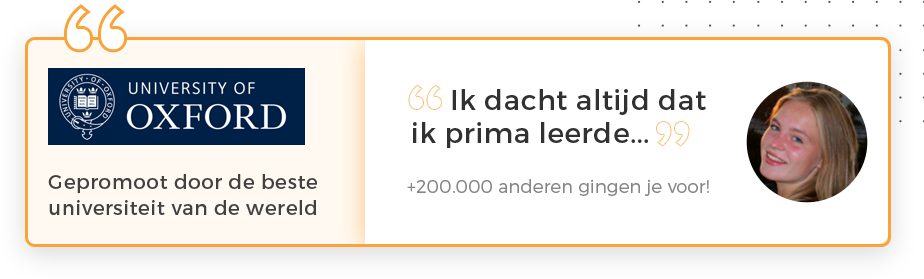# Samenvatting Risk management and financial institutions

ISBN-10 0138006172 ISBN-13 9780138006174
115 Flashcards en notities
3 Studenten

# Onthoud sneller, leer beter. Wetenschappelijk bewezen.## Dit is de samenvatting van het boek "Risk management and financial institutions". De auteur(s) van het boek is/zijn John Hull. Het ISBN van dit boek is 9780138006174 of 0138006172. Deze samenvatting is geschreven door studenten die effectief studeren met de studietool van Study Smart With Chris.

PREMIUM samenvattingen zijn gecontroleerd op kwaliteit en speciaal geselecteerd om je leerdoelen nog sneller te kunnen bereiken!• ## 1.1 LECTURE SHEETS SUMMARY LESSON 1&2

• What is Risk Management?

RM is a hybrid discipline combining tools of statistics, probability, economics, fincance, decision theory and management.

• What's the aim of RM?

- to identify, asess and prioritize risks;

- to minimize, monitor, and control the probability of unfortunate events;

• Name some of the benefits of RM to society

- it helps in lowering systemic risk;

- it aims at dispersing risks, so that economic shocks are more easily absorbed and do not cause cascading failures;

- it increases the general stability of the system.

• Name some of the benefits of RM to shareholders and companies.

- it may reduce tax costs, by reduing the variability of cash flow;

- it increases the credit scoring and the value of a company, by making bankruptcy less likely;

- it reduces the costs of external financing, since it helps in achieving optimal investment.

• What is risk? (definition restricted to economics and finance)

the probability that an actual return (or loss) on an investment/financial decision will be lower (or higher) than the expected return (or loss).

• Can we always quantify financial risks?

No, because of Knightian uncertainty (from Frank Knight). This is uncertainty that we cannot measure, calculate or estimate.

• Name three types of financial risk often discussed in the book.

Market Risk, Credit Risk and Operational Risk

• What is meant by the expected return?

The expected return is the expected value of the returns (or roughly: the weighted average of returns).

• Denote the formula for the standard deviation (STD(R)=...)

STD(R) = sqrt(E[R^2] - (E[R])^2)

• What's the practical use of a risk measure?

A risk measure is meant to determine the amount of an asset (or set of assets) to be kept in reserve. The aim of a reserve is to guarantee that the presence of capital that can be used as a (partial) cover if the risky event manifests itself, generating a loss.

• Denote the formula for the SD of a combined portfolio consisting of 2 investments.

sigma(portfolio) = sqrt ( w1^2*sig1^2 + w2^2*sig2^2 + 2*rho1,2*w1*w2*sig1*sig2 )

• What does the efficient frontier represent?

The EF represents all portfolios that maximize the E[R] and minimize sigma[R], thus dominating all other portfolios. The portfolios on the EF are convex linear combinations of all the portfolios of the economy.

• Give an example of a risk free invesment and explain why we call it 'risk free' and the difference between a risky investment.

An example of a risk free investment is a German federal bond (the bund). It's risk free because it guarantees a constant return (the risk free rate). The difference between the bund and a risky investment is that a risky investment has a higher expected return, but there's a chance it will generate a loss. The lower risk free return is 'sure'.

• How do we get from the efficient frontier to the new efficient frontier and what is represented by the touch point?

We add the Risk-free rate to the graph and then we draw a tangent line from this point to the EF. The point where the two meet represents the market portfolio.

• Which is a straight line: The new efficient frontier or the efficient frontier and why?

The NEF is a straight line. This is because we draw a tangent line from the risk-free rate to the EF and beyond, because now investors can choose the most rewarding portfolio combination in terms of the SD and return.

• What is the capital asset pricing model and give the formula's with and without alpha.

It's a model used to determine the theoretically appropriate required rate of return of an asset, if the asset is to be added to an already well-diversified portfolio, given that asset's non-diversifiable risk.

E[R] = alpha + beta*R(M) + epsilon

E[R] = (1-beta)*R(F) + beta*E[R(M)]

where beta*R(M) is known as systematic risk (SR) and epsilon is called the non-systematic/idyosincratic risk (IR).

• Explain the difference between systematic risk and non-systematic/idyosincratic risk.

IR can be controlled with diversification, but SR depends on the economy as a whole and can therefore not be diversified. E.g. a risk-averse investor will require extra returns to compensate for SR.

• Does the CAPM work in reality?

It is an estimation calculated with strong assumptions which don't apply to the real world (e.g. the world is guassian and linearity).  It's used because it's simple and easy to understand by non-experts.

Lees volledige samenvatting
Deze samenvatting. +380.000 andere samenvattingen. Een unieke studietool. Een oefentool voor deze samenvatting. Studiecoaching met filmpjes.